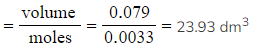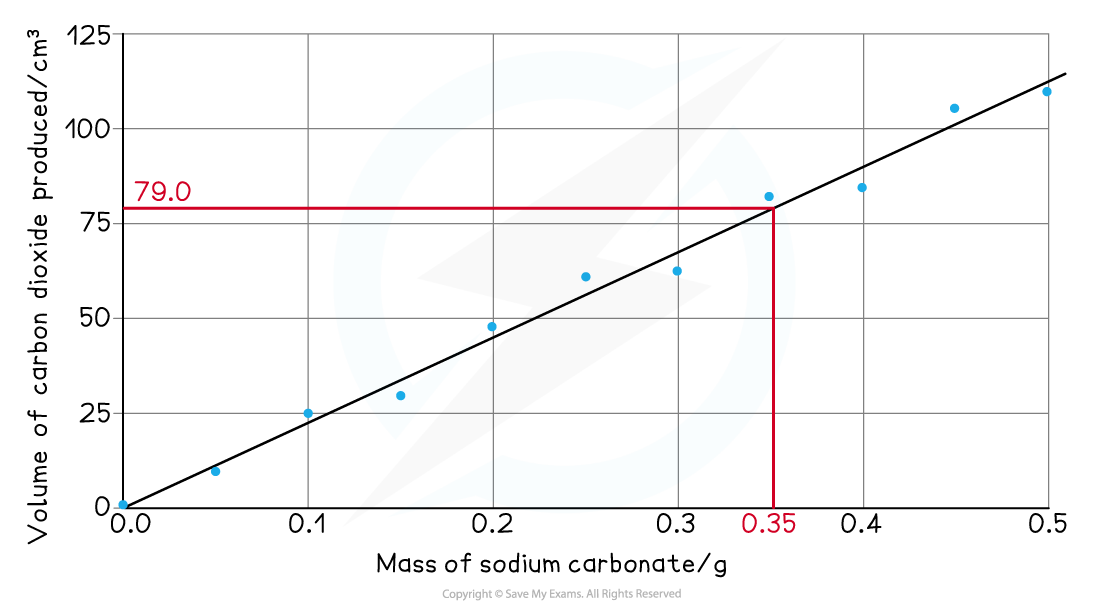# Edexcel A Level Chemistry:复习笔记4.1.1 Molar Volume of a Gas

### Core Practical 1: Measuring the Molar Volume of a Gas

#### Measuring gas volumes

• The volume of gas produced in a reaction can be measured by collecting the gas with a gas syringe or by the displacement of water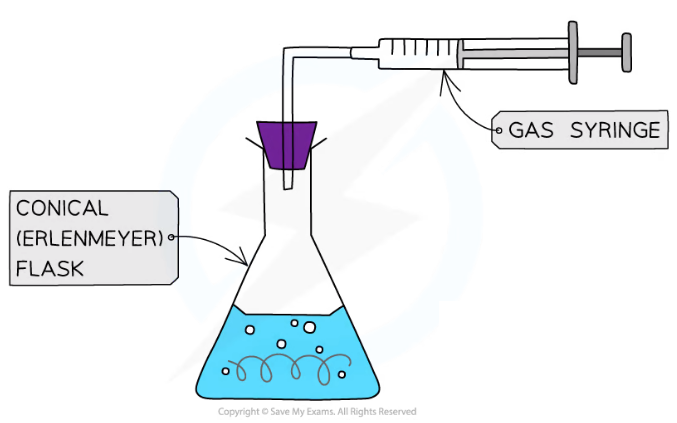Gas syringe equipment for collecting the gas produced in a reactionDisplacement of water equipment for collecting the gas produced in a reaction

Sample method

• For the reaction of hydrochloric acid and sodium carbonate

Na2CO3 (s) + 2HCl (aq) → 2NaCl (aq) + H2O (l) + CO2 (g)

1. Measure out a fixed volume of hydrochloric acid, e.g. 25.0 cm3, into a conical flask
2. Add a known mass of sodium carbonate, e.g. 0.05 g, to the conical flask
3. Immediately connect the gas syringe delivery tube
4. Allow the reaction to go to completion
5. Record the volume of carbon dioxide produced
6. Repeat the experiment with different masses of sodium carbonate, e.g. 0.10 g, 0.15 g, 0.20 g, 0.25 g... 0.50 g
7. Some assumptions are made about the experiment:
• The amount of gas lost between adding the sodium carbonate and connecting the delivery tube is negligible
• The delivery tube set up is airtight so no gas is lost
• The reaction does go to completion

#### Sample results

Mass volume results table

####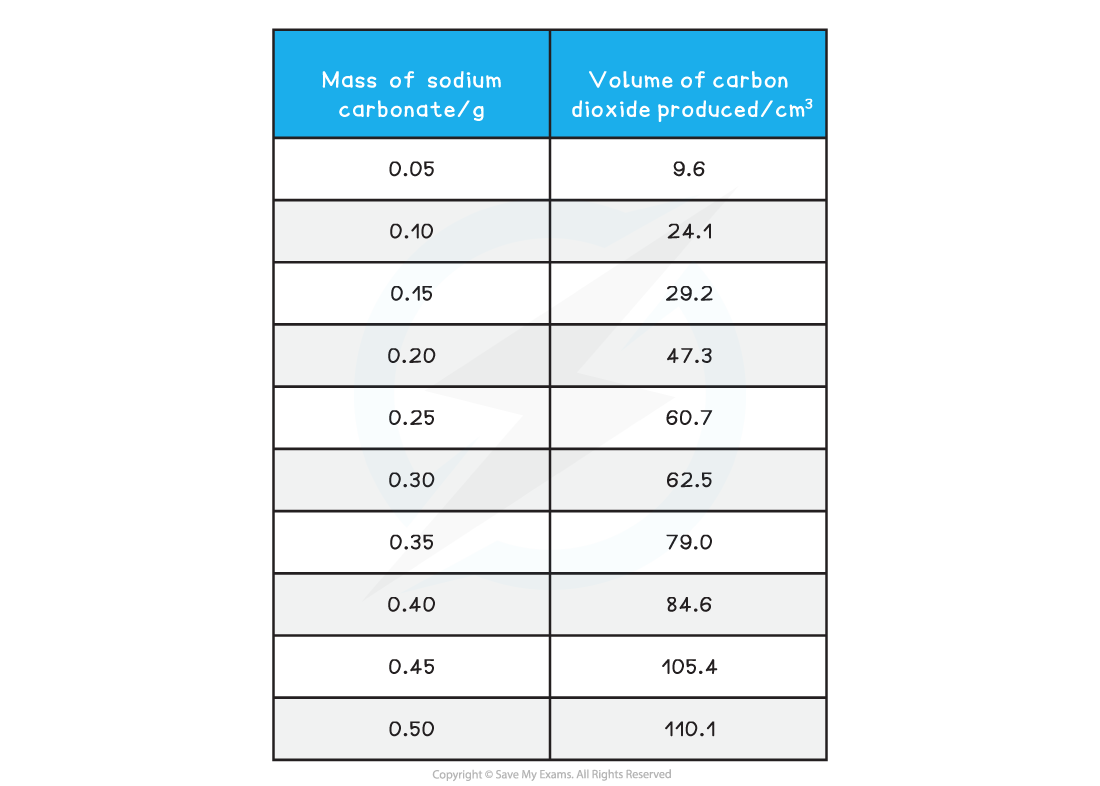• The results are then plotted on to a graph
• Mass of sodium carbonate on the x-axis and volume or carbon dioxide produced on the y-axis
• Anomalous results are ignored and one straight line (or one smooth curve) of best fit is added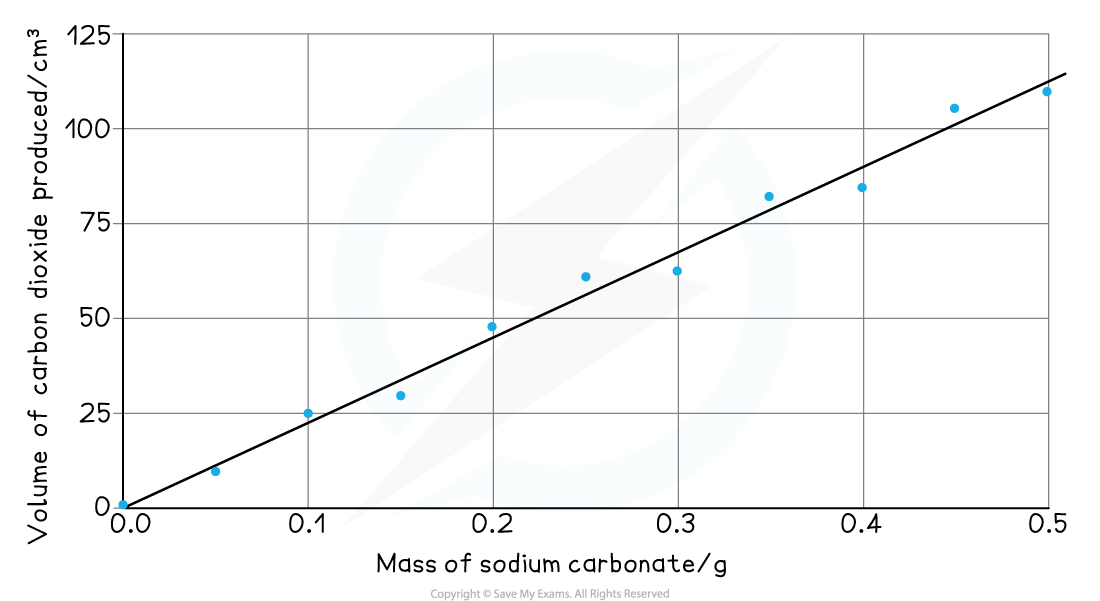#### Analysis

• Read off the volume of gas produced for a sensible mass of sodium carbonate, e.g. 0.35 g produces 79.0 cm3
• The mass of sodium carbonate may be specified in an exam question

Na2CO3 (s) + 2HCl (aq) → 2NaCl (aq) + H2O (l) + CO2 (g)

• From the reaction equation, one mole of sodium carbonate produces one mole of carbon dioxide
• Calculate the molar mass of sodium carbonate
• (2 x 23.0) + 12.0 + (3 x 16.0) = 106.0
• Calculate the number of moles of sodium carbonate, using the mass from your graph reading
• Moles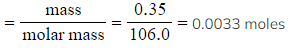• Convert the volume of carbon dioxide from your graph reading from cm3 to dm3
•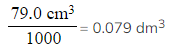• Calculate the molar volume of gas produced:
• Molar gas volume